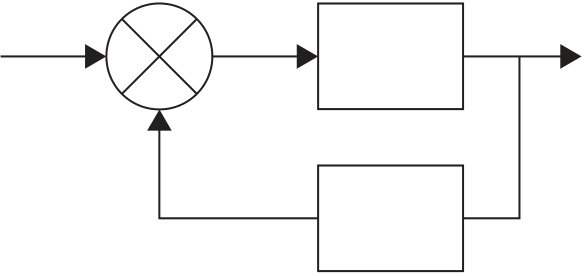Rating : ⭐⭐⭐⭐⭐
Price : \$10.99
Language:EN
Pages: 2

# Chapter outputg system with negative feedbackan output824

Chapter 18

Input
Error
Feedback

Figure 18.101 : System with negative feedback

an output of H(s)Y(s). Thus the feedback signal is H(s)Y(s). The error is the difference between the system input signal X(s) and the feedback signal and is thus:

X s ( )
G s ( )
X s ( ) H s Y s ( ) ( )

and so:

[ 1� G s H s Y s

G s X s ( ) ( )

Y s ( )
X s ( ) 1 G s H s ( )

For a system with positive feedback ( Figure 18.102 ), the feedback signal is H(s)Y(s) and thus the input to the G(s) system is X(s) �H(s)Y(s). Hence:

G s ( )

Y s ( )

X s ( ) H s Y s ( ) ( )
[ 1� G s H s Y s
www.newnespress.com
Control and Instrumentation Systems
Input

Error

G(s)
X(s) Y(s)
Feedback
Input 2
X(s) s � 2

Y(s)

4

overall transfer function � Y s ( )
X s ( ) 1 G s H s ( )

Example 18.18
Determine the overall transfer function for a control system ( Figure 18.103 ) which has a negative feedback loop with a transfer function 4 and a forward path transfer function of 2 / ( s� 2).

Solution
The overall transfer function of the system is:

s 2 2
1 4 2 s 10
s 2
www.newnespress.com
How It Works